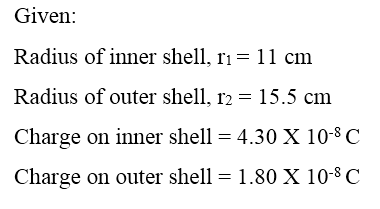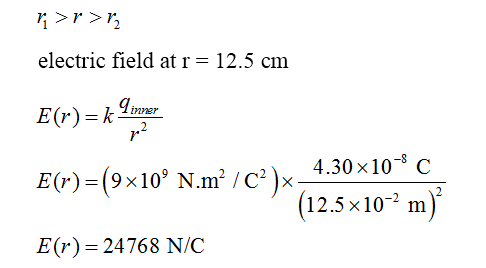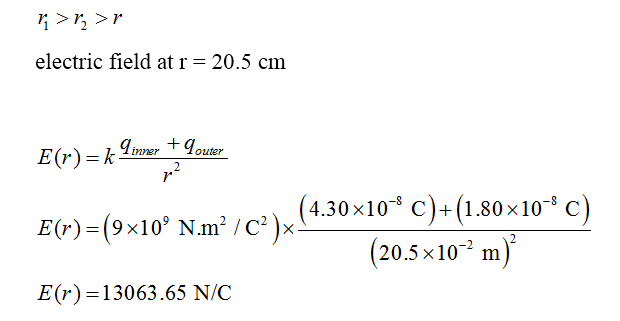# Two charged concentric spherical shells have radii of 11.0 cm and 15.5 cm. The charge on the inner shell is 4.30 ✕ 10−8 C and that on the outer shell is 1.80 ✕ 10−8 C. Find the magnitude of the electric field at the following points.(a) at r = 12.5 cm _____________N/C(b) at r = 20.5 cm _____________N/C(please show the units so that I can follow along easier)

Question
18 views

Two charged concentric spherical shells have radii of 11.0 cm and 15.5 cm. The charge on the inner shell is 4.30 ✕ 10−8 C and that on the outer shell is 1.80 ✕ 10−8 C. Find the magnitude of the electric field at the following points.

(a) at r = 12.5 cm
_____________N/C

(b) at r = 20.5 cm
_____________N/C

check_circle

Step 1Step 2Step 3...

### Want to see the full answer?

See Solution

#### Want to see this answer and more?

Solutions are written by subject experts who are available 24/7. Questions are typically answered within 1 hour.*

See Solution
*Response times may vary by subject and question.
Tagged in